MSAE 530
Binary Phase Diagram I2
Overview of this lecture
How to define phase equilibrium?
Characteristics of miscibility gap
Characteristics of regular solution at critical
temperature
Examples of real solutions
Gibbs’ Phase Rule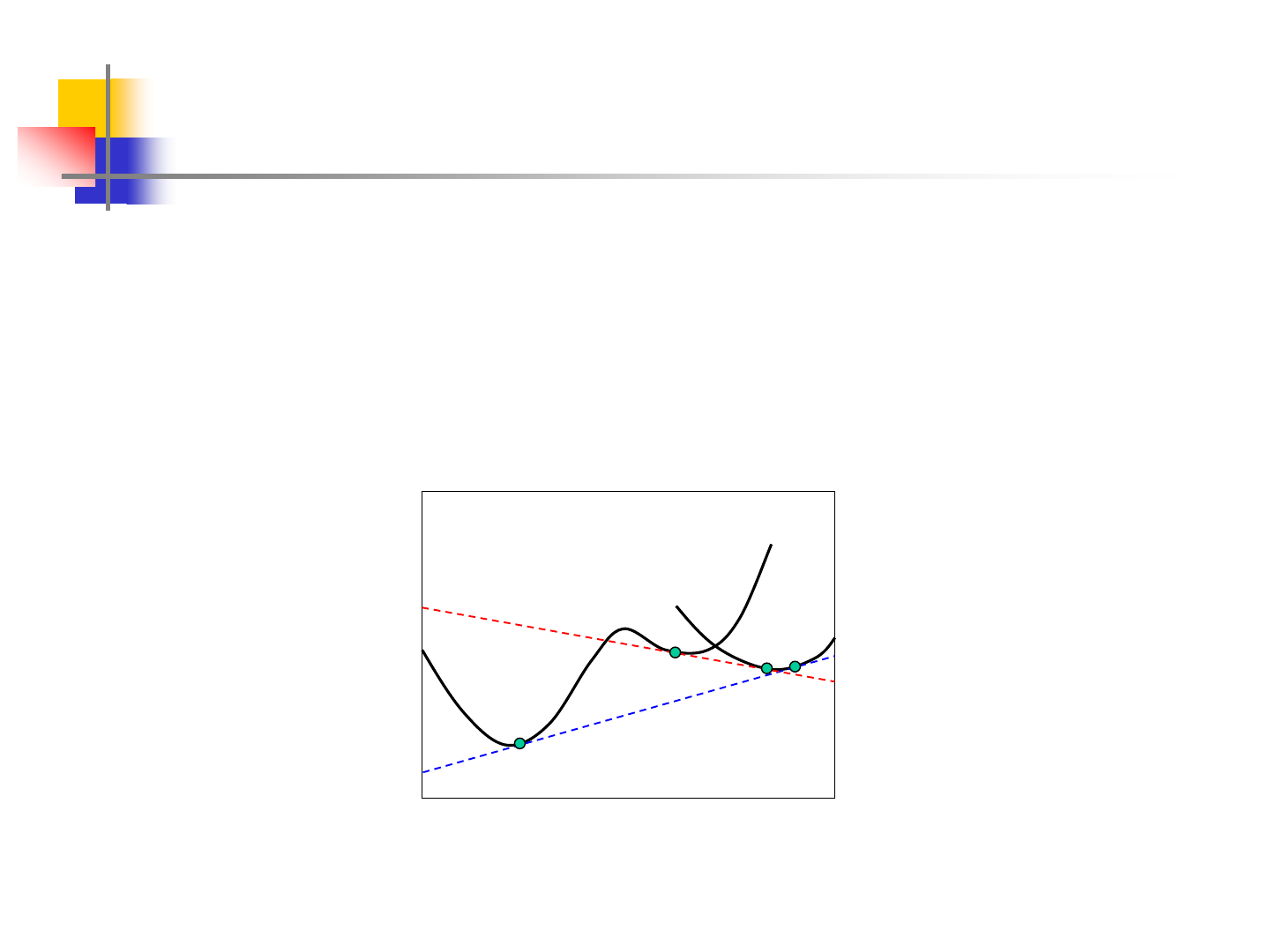3
Introduction
A minimum in G, in the case of a mixture of phases, corresponds
with the equilibrium condition given by:
components allfor
III
i
II
i
I
i
==
I
II
A B
mol fracn. B
G
a
b
c
d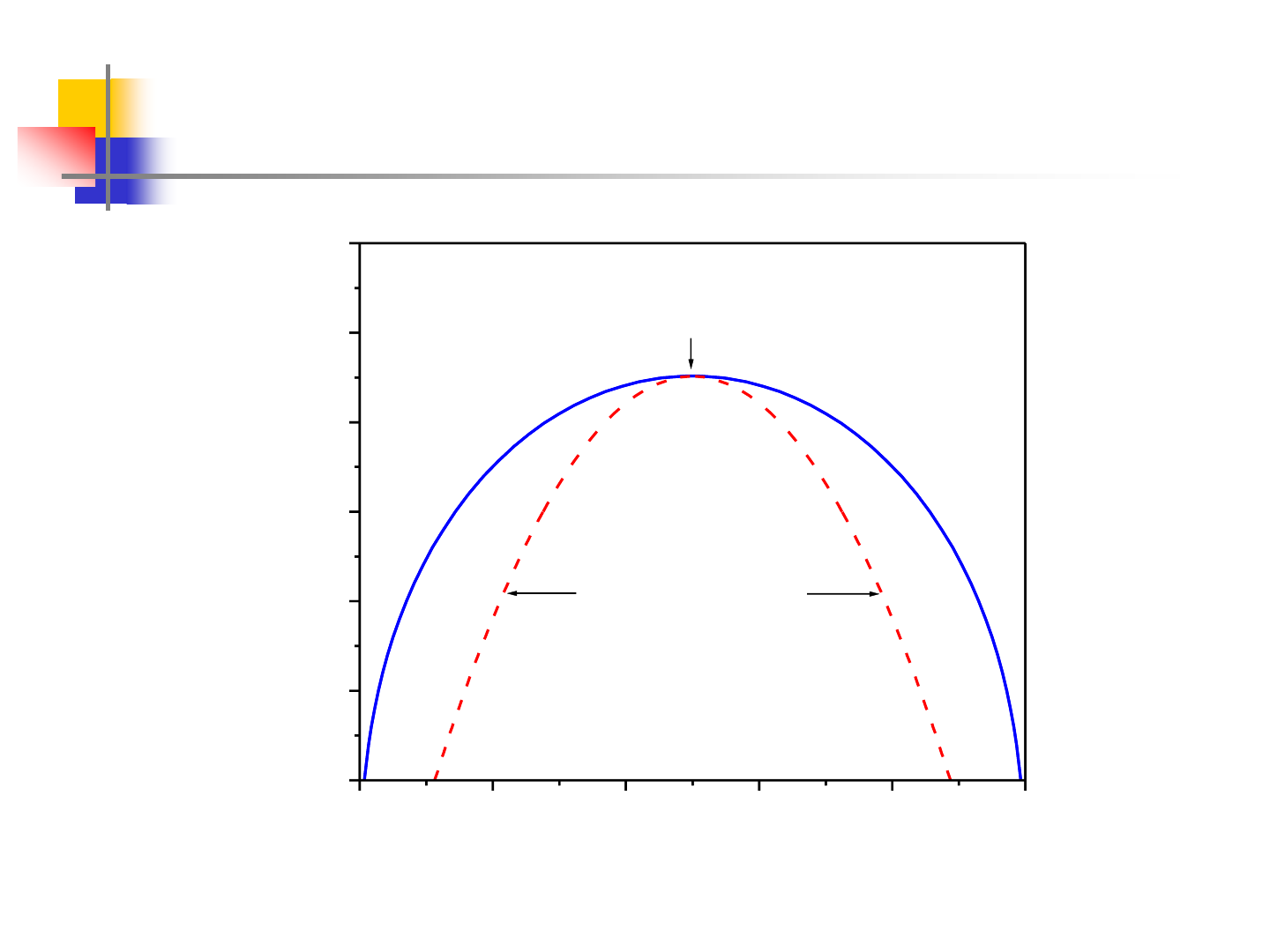4
Miscibility Gap
0.0 0.2 0.4 0.6 0.8 1.0
300
400
500
600
700
800
900
critical point
1
+
2
2
1
=12500 J/mol
B
A
T(K)
x
B
metastable
metastable
Spinodal line
unstable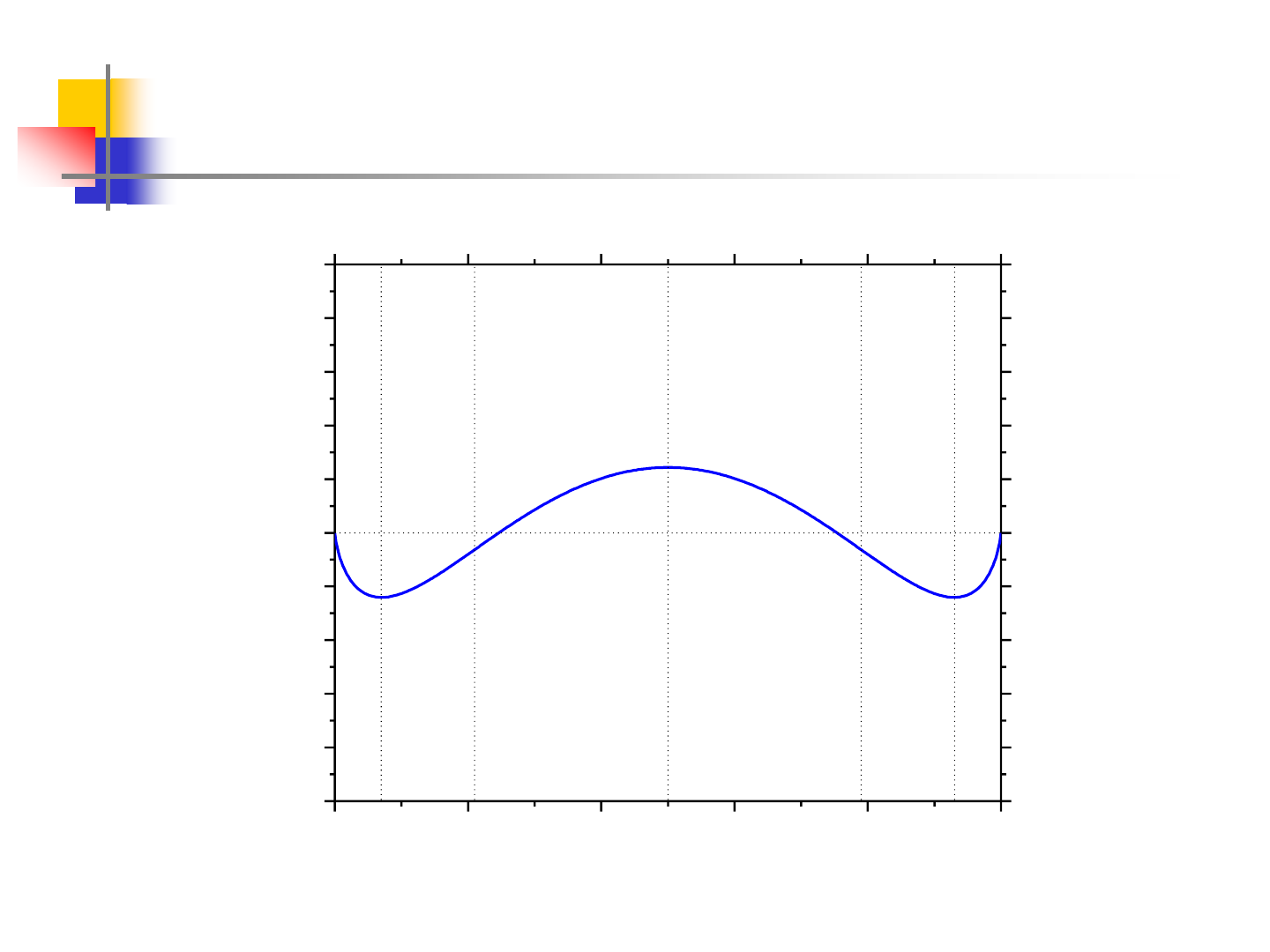5
Miscibility Gap at 500K
0.0 0.2 0.4 0.6 0.8 1.0
-1.0
-0.8
-0.6
-0.4
-0.2
0.0
0.2
0.4
0.6
0.8
1.0
0.0 0.2 0.4 0.6 0.8 1.0
-1.0
-0.8
-0.6
-0.4
-0.2
0.0
0.2
0.4
0.6
0.8
1.0
e
d
c
b
a
T=500K
B
A
G(kJ/mol)
X
2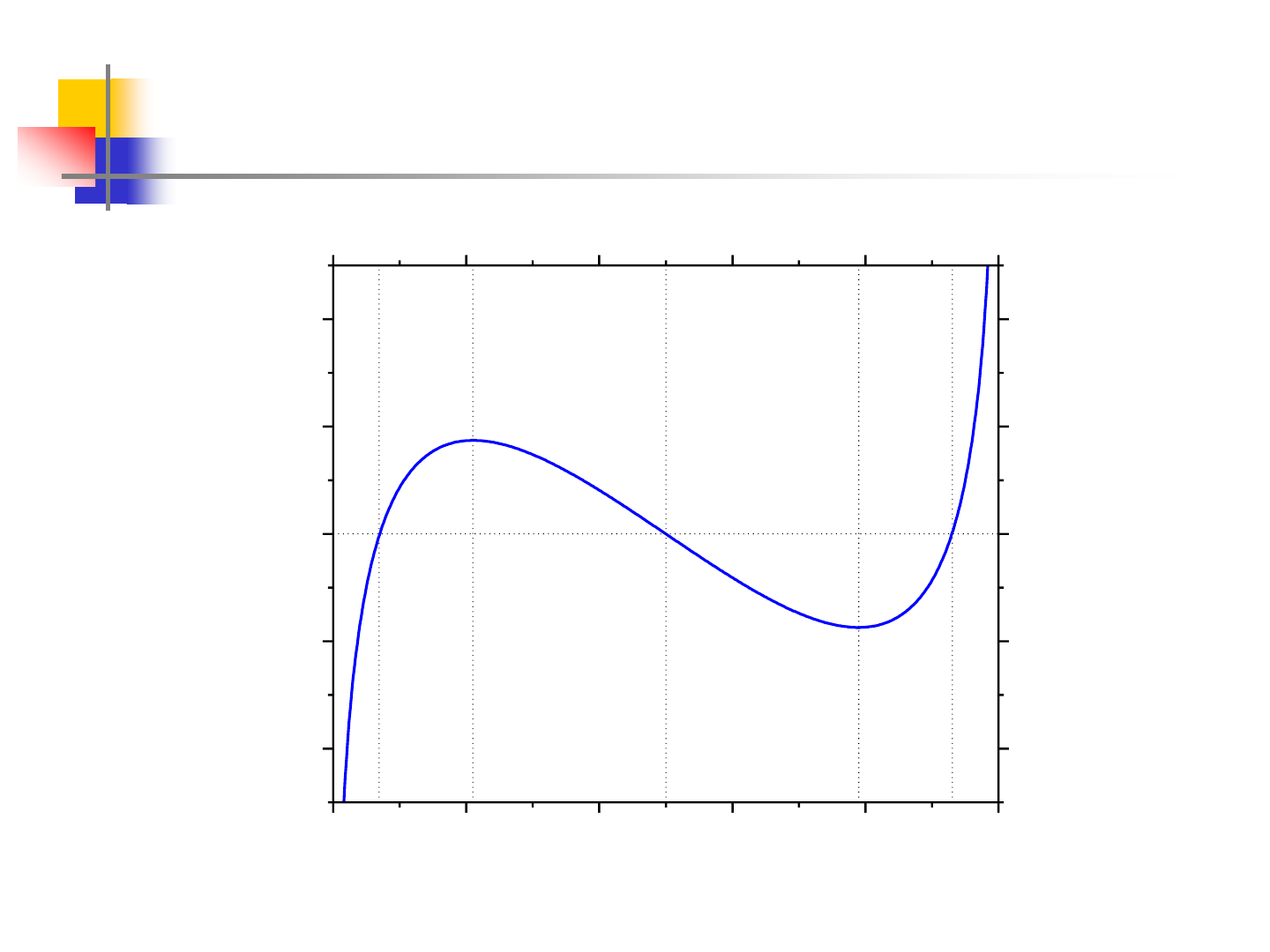6
Miscibility Gap at 500K
0.0 0.2 0.4 0.6 0.8 1.0
-4
-2
0
2
4
0.0 0.2 0.4 0.6 0.8 1.0
-4
-2
0
2
4
ed
c
b
a
B
A
T=500K
dG/dx (kJ/mol)
x
2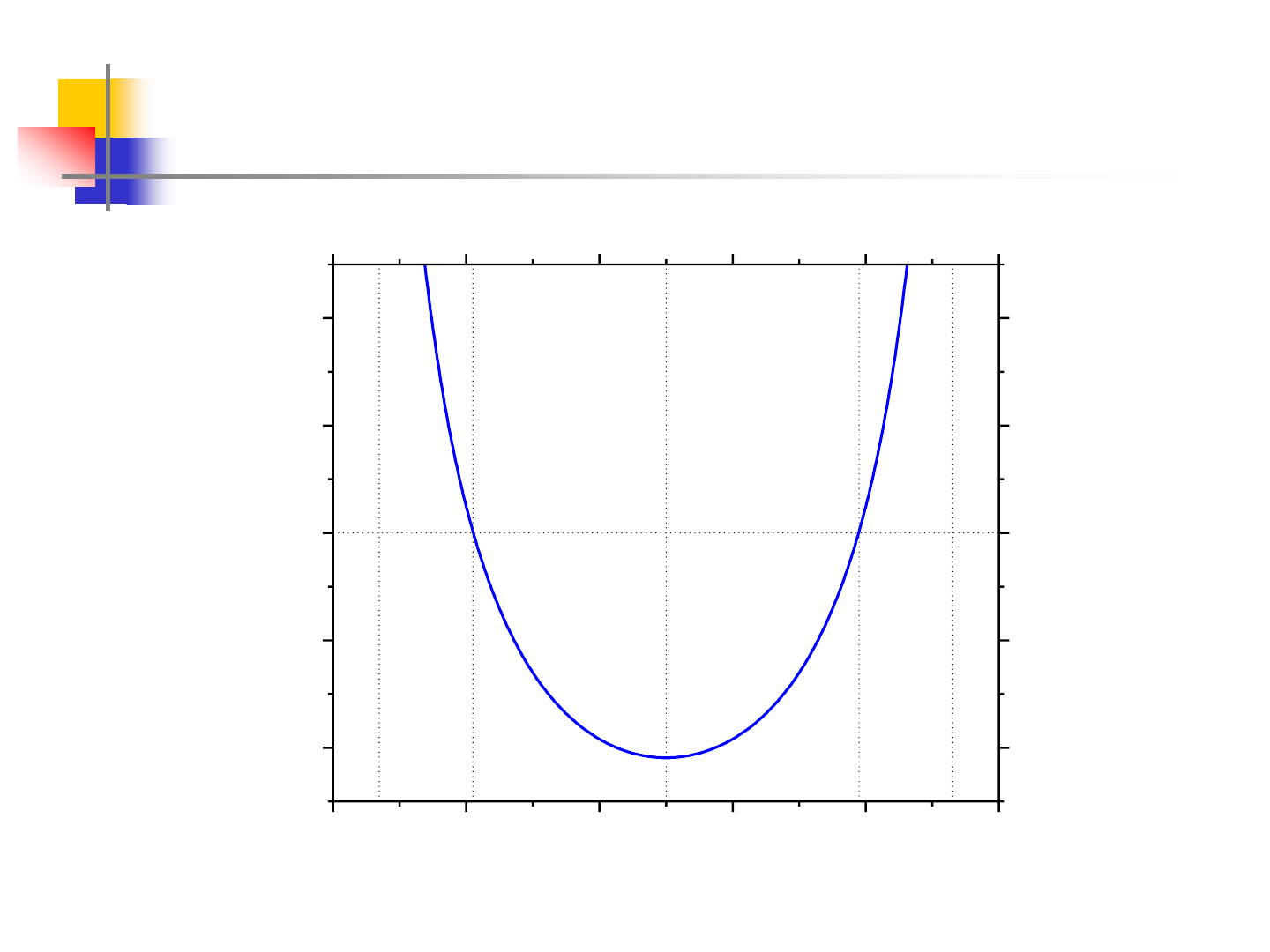7
Miscibility Gap at 500K
0.0 0.2 0.4 0.6 0.8 1.0
-8
-4
0
4
8
0.0 0.2 0.4 0.6 0.8 1.0
-8
-4
0
4
8
T=500K
e
b
c d
a
B
A
d
2
G/dx
2
(kJ/mol)
x
2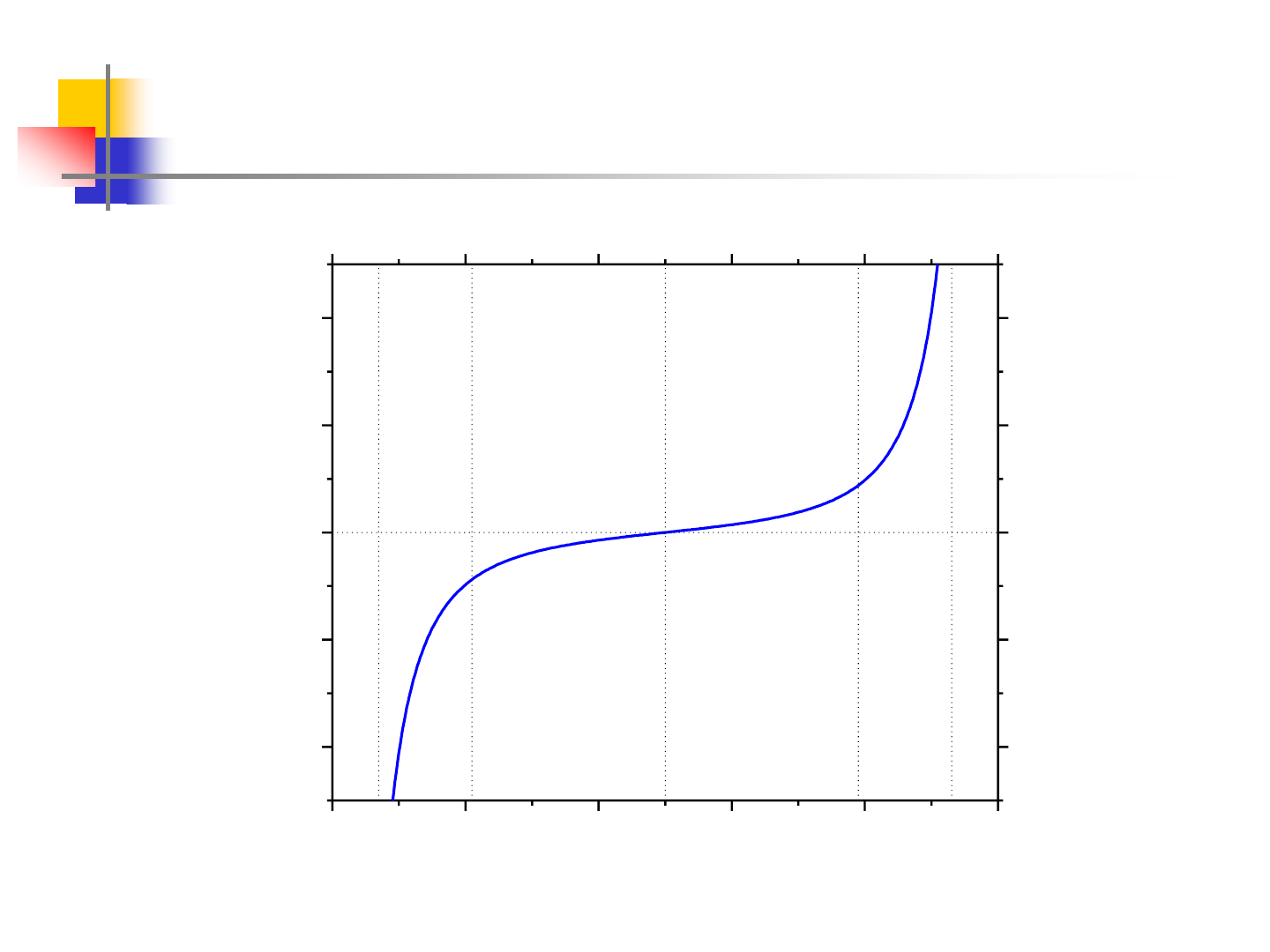8
Miscibility Gap at 500K
0.0 0.2 0.4 0.6 0.8 1.0
-400
-200
0
200
400
0.0 0.2 0.4 0.6 0.8 1.0
-400
-200
0
200
400
b
c d
e
a
T=500K
B
A
d
3
G/dx
3
(kJ/mol)
x
2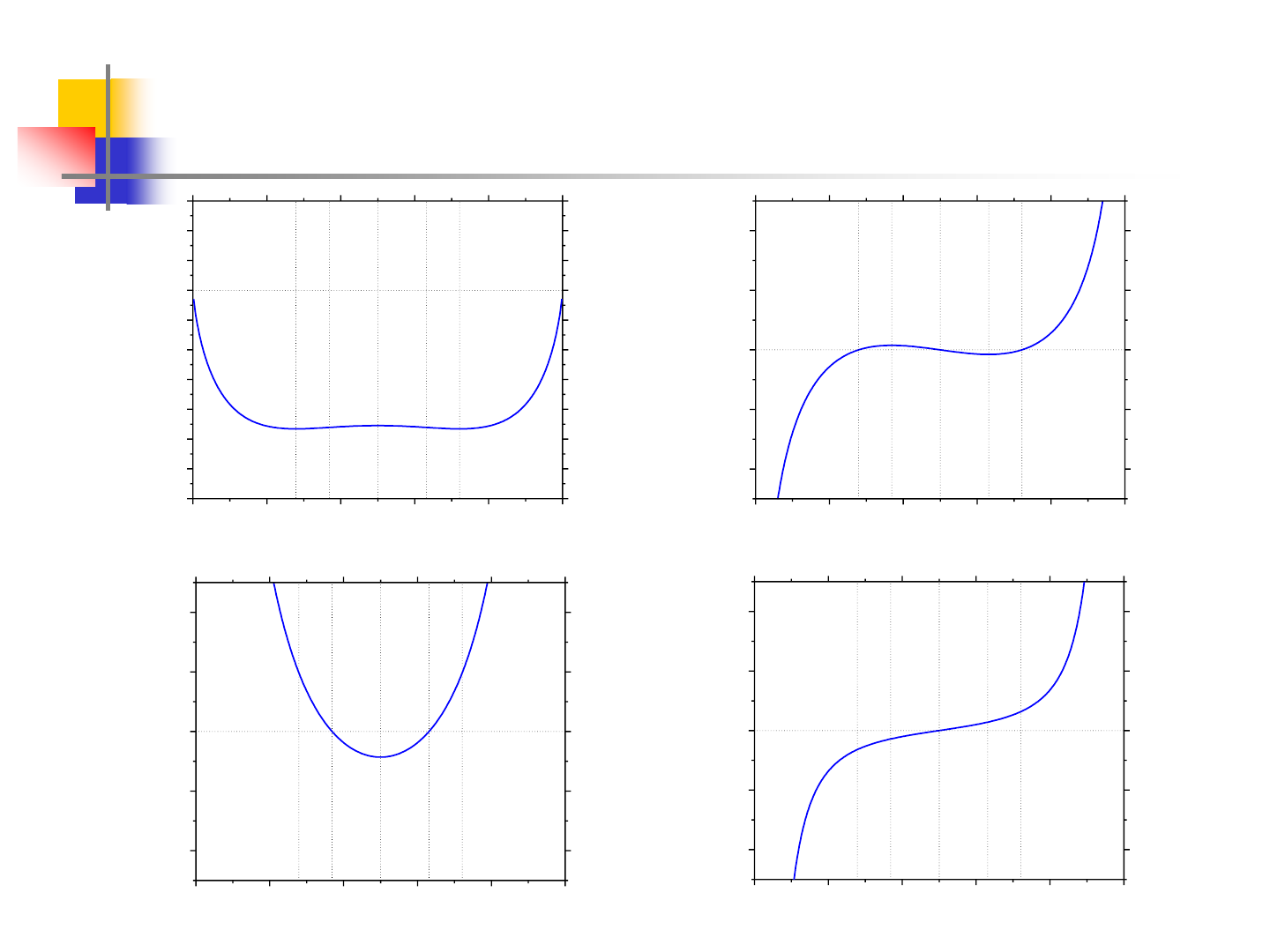9
Miscibility Gap at 700K
0.0 0.2 0.4 0.6 0.8 1.0
-1.4
-1.2
-1.0
-0.8
-0.6
-0.4
-0.2
0.0
0.2
0.4
0.6
0.0 0.2 0.4 0.6 0.8 1.0
-1.4
-1.2
-1.0
-0.8
-0.6
-0.4
-0.2
0.0
0.2
0.4
0.6
e
d
c
b
a
T=700K
B
A
G(kJ/mol)
X
2
0.0 0.2 0.4 0.6 0.8 1.0
-8
-4
0
4
8
0.0 0.2 0.4 0.6 0.8 1.0
-8
-4
0
4
8
T=700K
e
b
c d
a
B
A
d
2
G/dx
2
(kJ/mol)
x
2
0.0 0.2 0.4 0.6 0.8 1.0
-400
-200
0
200
400
0.0 0.2 0.4 0.6 0.8 1.0
-400
-200
0
200
400
b
c d ea
T=700K
B
A
d
3
G/dx
3
(kJ/mol)
x
2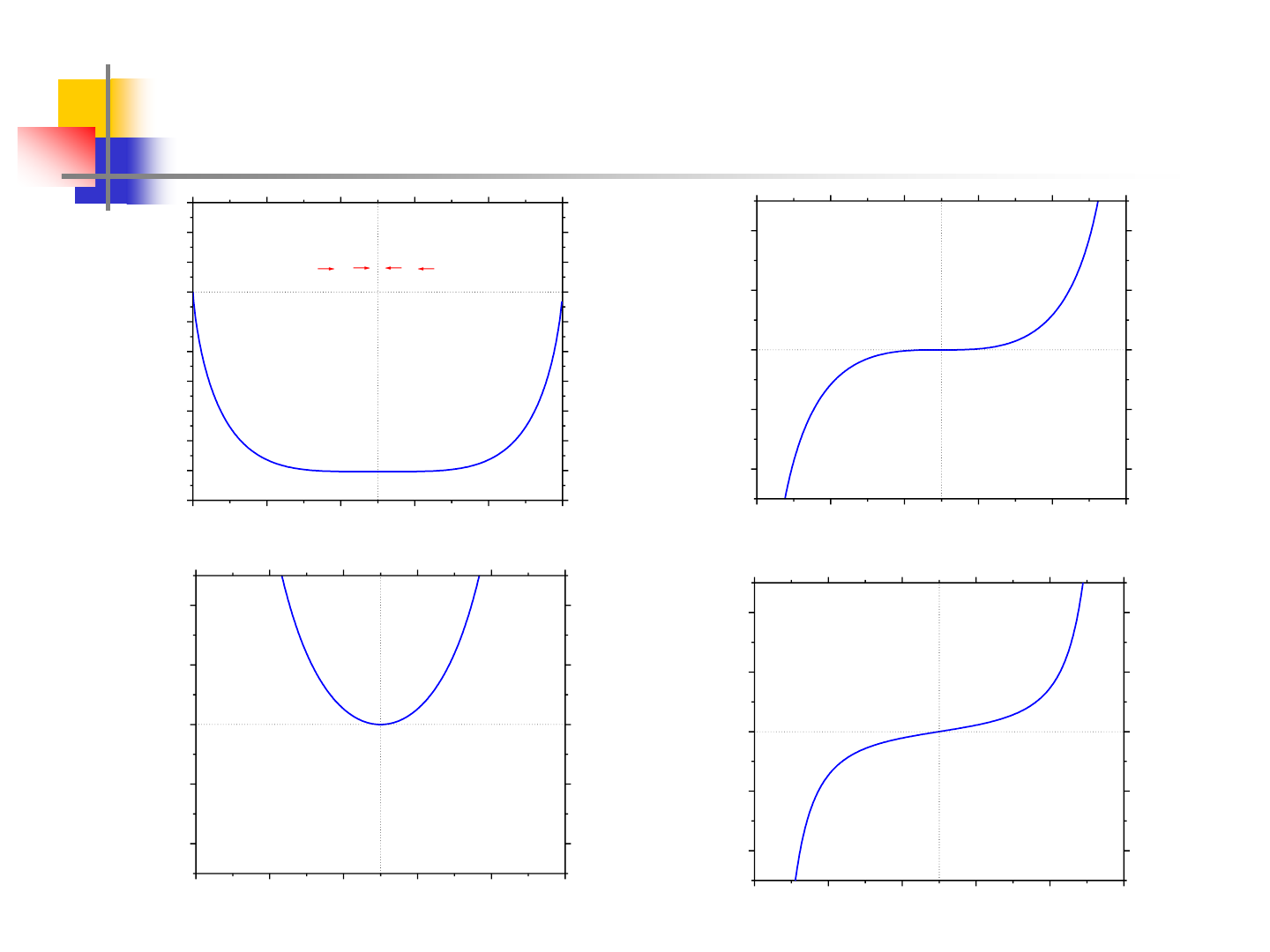10
Miscibility Gap at 751.7K
0.0 0.2 0.4 0.6 0.8 1.0
-1.4
-1.2
-1.0
-0.8
-0.6
-0.4
-0.2
0.0
0.2
0.4
0.6
0.0 0.2 0.4 0.6 0.8 1.0
-1.4
-1.2
-1.0
-0.8
-0.6
-0.4
-0.2
0.0
0.2
0.4
0.6
e
d
c
b
a
T=Tc=751.7K
B
A
G(kJ/mol)
X
2
0.0 0.2 0.4 0.6 0.8 1.0
-8
-4
0
4
8
0.0 0.2 0.4 0.6 0.8 1.0
-8
-4
0
4
8
T=Tc=751.7K
B
A
d
2
G/dx
2
(kJ/mol)
x
2
0.0 0.2 0.4 0.6 0.8 1.0
-400
-200
0
200
400
0.0 0.2 0.4 0.6 0.8 1.0
-400
-200
0
200
400
T=Tc=751.7K
B
A
d
3
G/dx
3
(kJ/mol)
x
2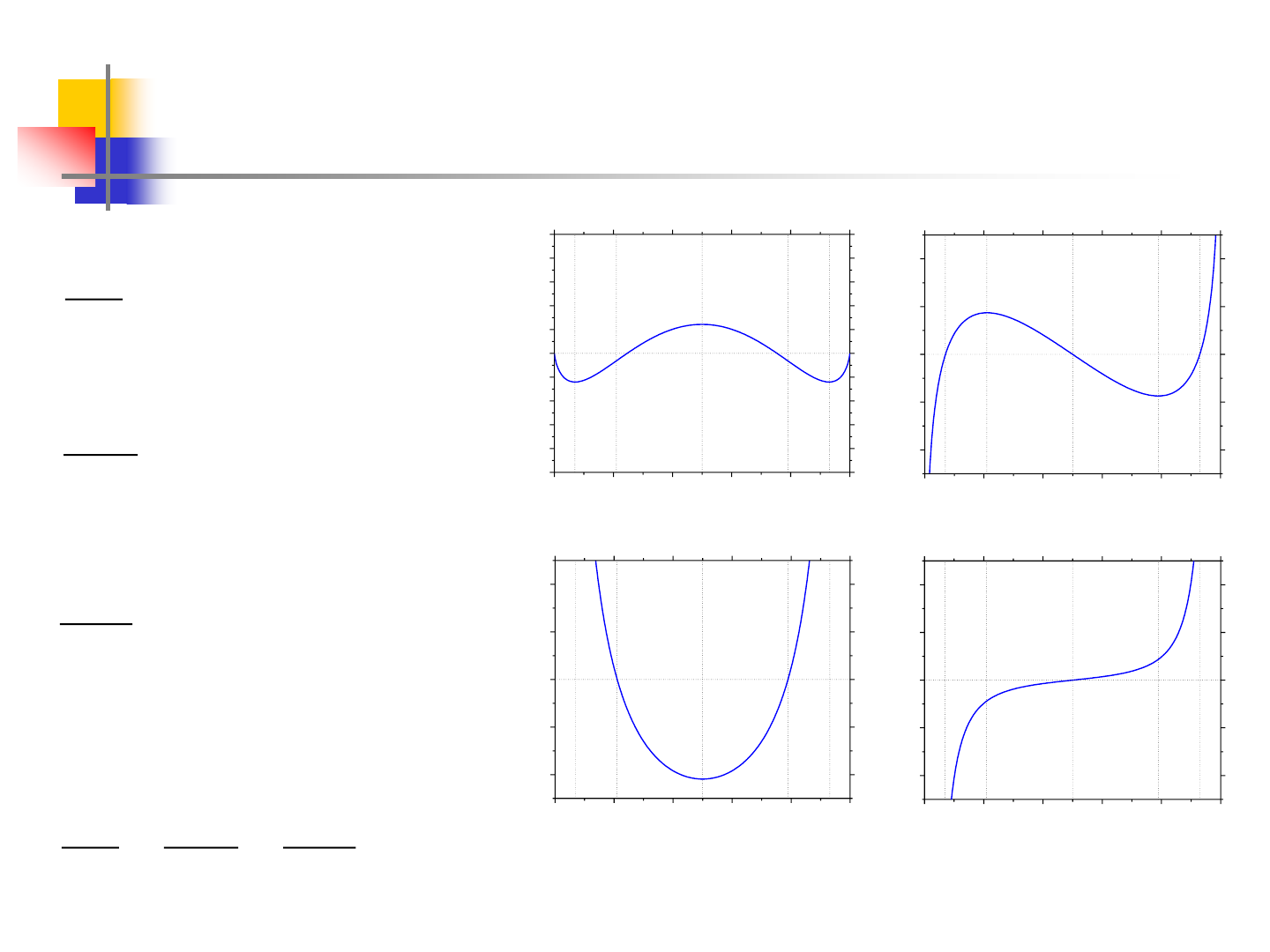11
Miscibility Gap - Regular Solution
0.0 0.2 0.4 0.6 0.8 1.0
-1.0
-0.8
-0.6
-0.4
-0.2
0.0
0.2
0.4
0.6
0.8
1.0
0.0 0.2 0.4 0.6 0.8 1.0
-1.0
-0.8
-0.6
-0.4
-0.2
0.0
0.2
0.4
0.6
0.8
1.0
e
d
c
b
a
T=500K
B
A
G(kJ/mol)
X
2
0.0 0.2 0.4 0.6 0.8 1.0
-4
-2
0
2
4
0.0 0.2 0.4 0.6 0.8 1.0
-4
-2
0
2
4
ed
c
b
a
B
A
T=500K
dG/dx (kJ/mol)
x
2
0.0 0.2 0.4 0.6 0.8 1.0
-8
-4
0
4
8
0.0 0.2 0.4 0.6 0.8 1.0
-8
-4
0
4
8
T=500K
e
b
c d
a
B
A
d
2
G/dx
2
(kJ/mol)
x
2
0.0 0.2 0.4 0.6 0.8 1.0
-400
-200
0
200
400
0.0 0.2 0.4 0.6 0.8 1.0
-400
-200
0
200
400
b
c d
e
a
T=500K
B
A
d
3
G/dx
3
(kJ/mol)
x
2
eca
x, x,at x 0
dx
dG
=
db
2
2
x,at x 0
dx
Gd
=
c
3
3
at x 0
dx
Gd
=
c
3
3
2
2
C
at x 0
dx
Gd
dx
Gd
dx
dG
TT as
===12
Miscibility Gap Regular Solution
id
TSx)1εx(G =
x1
x
lnRTx)21ε(
dx
dG
+=
++=
x1
1
x
1
RTε2
dx
Gd
2
2
223
3
)x1(x
1x2
RT
dx
Gd
=
At T = T
C
, x = x
C
0
)x1(x
1x2
,0
dx
Gd
2
C
2
C
C
3
3
=
=
X
C
= 0.5
+==
CC
C
2
2
x1
1
x
1
RT2 ,0
dx
Gd
= 2RT
C
phases! existing-co theof nscompositio obtain the toused becan 0
dx
dG
=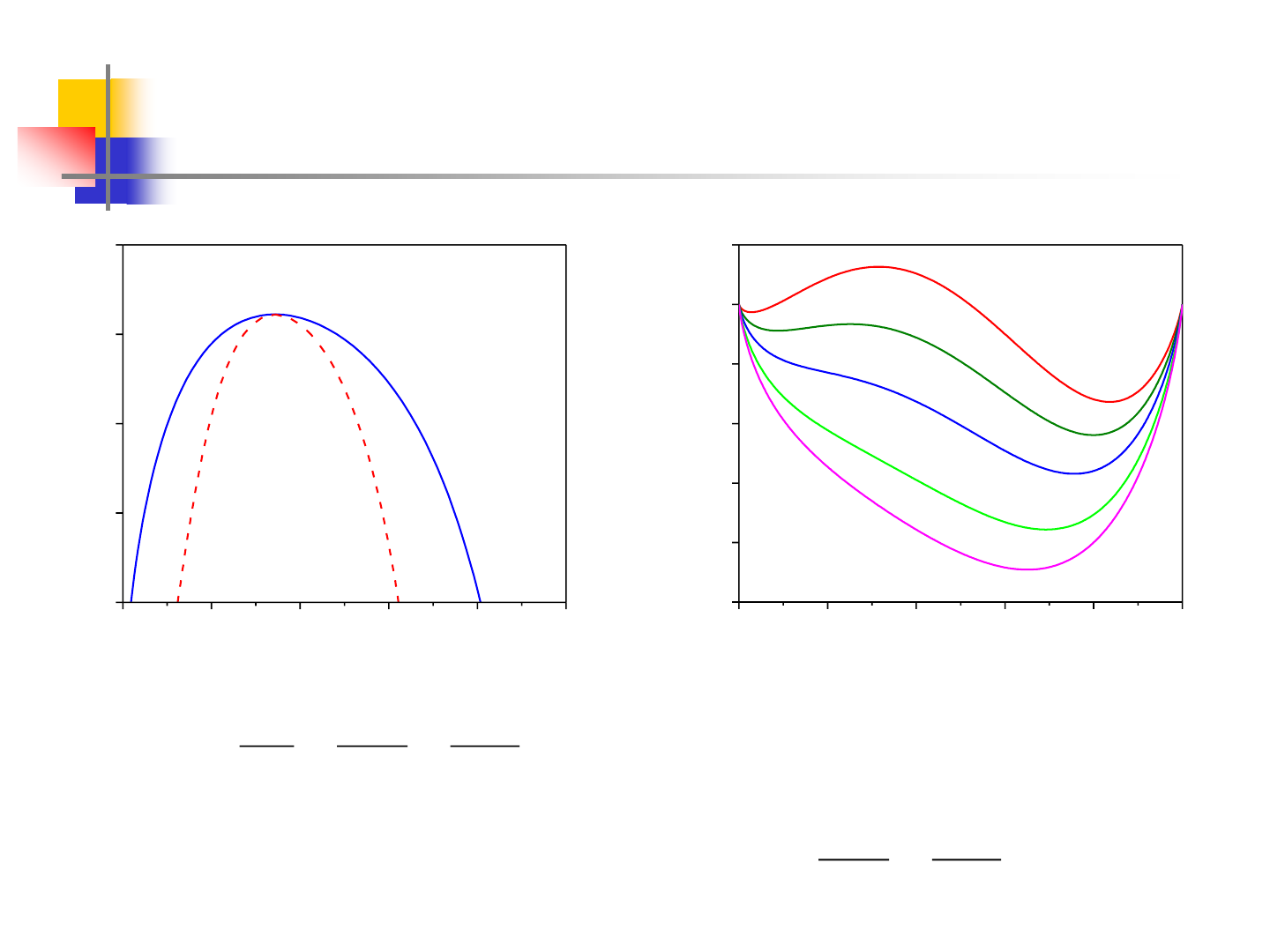13
Miscibility Gap Real Example
0.0 0.2 0.4 0.6 0.8 1.0
300
400
500
600
700
Tc = 622.3K
Xc = 0.344
2
1
Zn
Al
T / K
mol. fracn. Zn
0.0 0.2 0.4 0.6 0.8 1.0
-2.5
-2.0
-1.5
-1.0
-0.5
0.0
0.5
700K
622.3K
500K
400K
300K
ZnAl
mix
G (kJ/mol)
mol. fracn. Zn
holds. 0
dx
Gd
dx
Gd
only solutions,regular -sub-subor regular -sub
for but solution,regular for holds at x 0
dx
Gd
dx
Gd
dx
dG
,TT As
3
3
2
2
c
3
3
2
2
C
==
===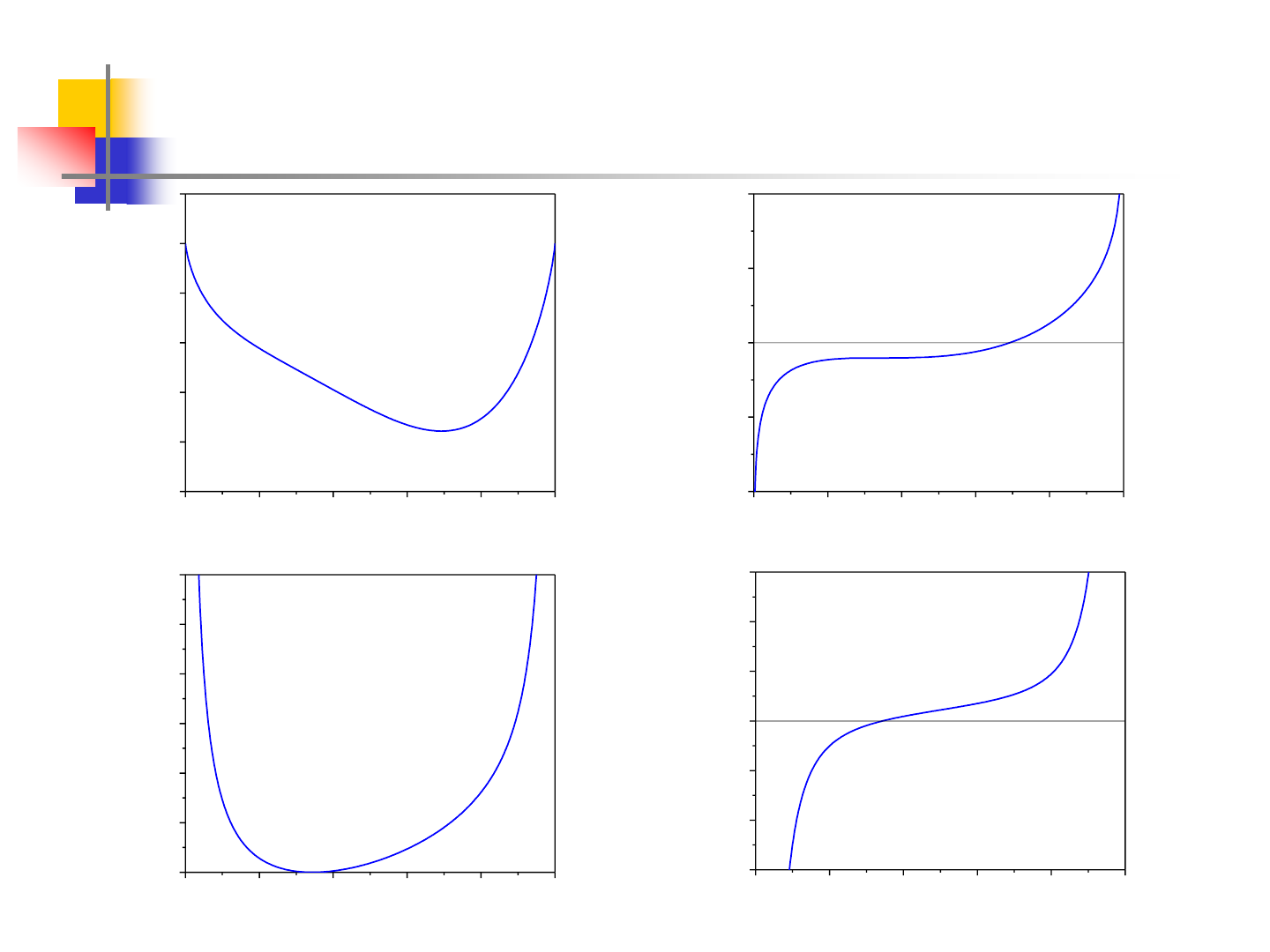14
Miscibility Gap Real Example
0.0 0.2 0.4 0.6 0.8 1.0
-2.5
-2.0
-1.5
-1.0
-0.5
0.0
0.5
T=Tc=622.3K
ZnAl
mix
G (kJ/mol)
mol. fracn. Zn
0.0 0.2 0.4 0.6 0.8 1.0
-20
-10
0
10
20
x
Zn
Zn
Al
dG/dx (kJ/mol)
0.0 0.2 0.4 0.6 0.8 1.0
0
20
40
60
80
100
120
Zn
Al
d
2
G/dx
2
(kJ/mol)
x
Zn
0.0 0.2 0.4 0.6 0.8 1.0
-600
-400
-200
0
200
400
600
Zn
Al
x
Zn
dG
3
/dx
3
(kJ/mol)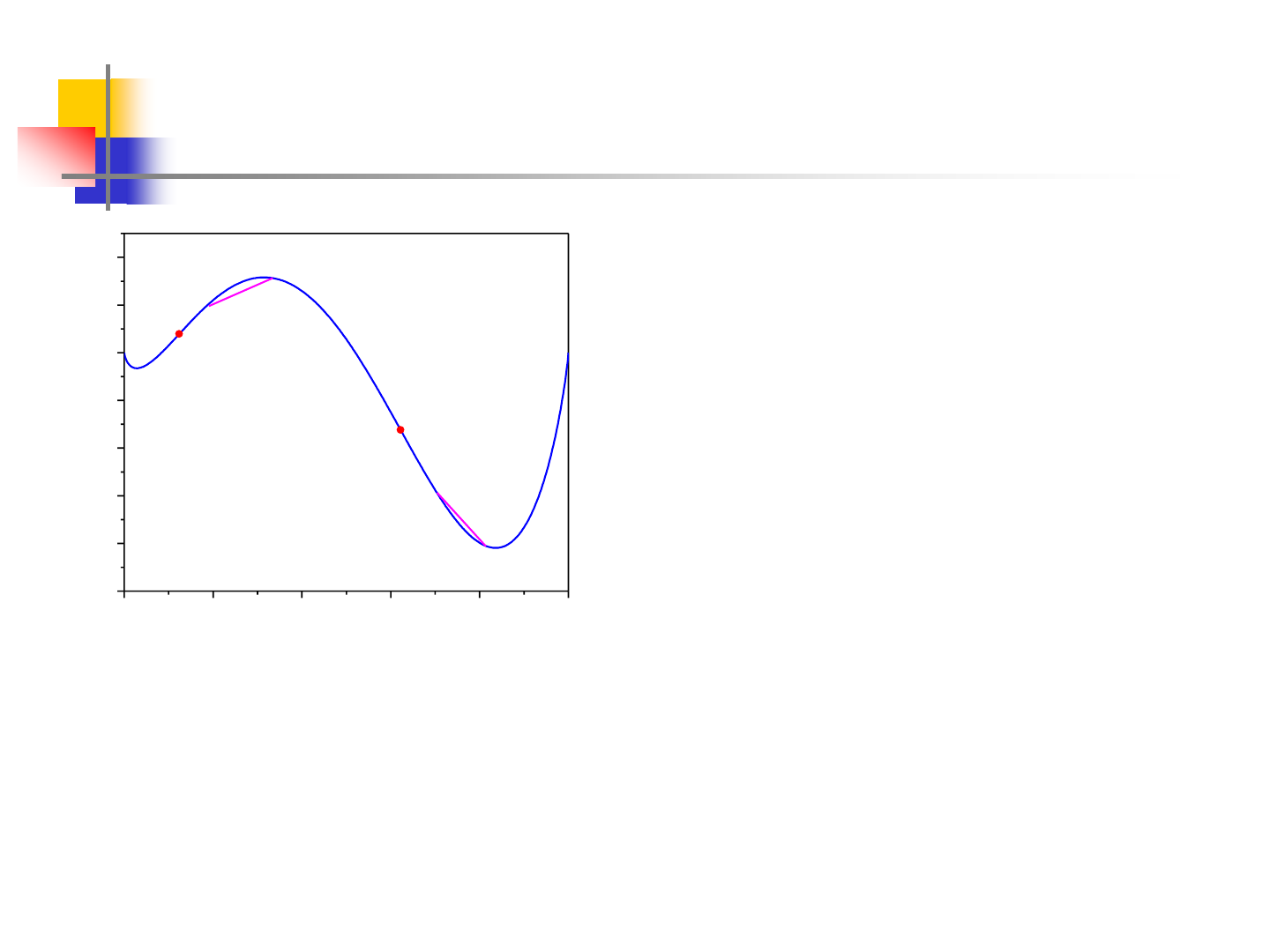15
Chemical Spinodal
0.0 0.2 0.4 0.6 0.8 1.0
-1.0
-0.8
-0.6
-0.4
-0.2
0.0
0.2
0.4
b
a
d
c
S2
S1
T=300K
Zn
Al
mix
G (kJ/mol)
mol. fracn. Zn
(a) Between the spinodal compositions,
the Gibbs energy of homogenous single-
phase alloy is always higher than that of
any mixture of compositions (c and d,
for example), which can form from a
decomposition of this phase.
(b) Between the miscibility gap and the nearby spinodal composition,
the Gibbs energy will increase if a single-phase alloy decomposed
locally to form any mixture of compositions(a and b, for example).
Decomposition can only take place by nucleation of the other phase.16
Gibbs’ Phase Rule
The Phase Rule is concerned with evaluating the number of
independent intensive variables (degrees of freedom) required to
specify a state, i.e. the different features of a phase diagram.
I. Total number of variables
Total number of variables for each phase: C+2
Total number of variables for phases: (C+2)
II. Constraints
(a) T
(1)
= T
(2)
= … = T
()
(-1) relations
(b) p
(1)
= p
(2)
= … = p
()
(-1) relations
(c)
I
(j)
constraints R
relations17
Gibbs’ Phase Rule
III. Number of independent variables, F
F = C + 2 - R
p-V work only
F = C + 1 - R
p-V work only, at constant p
When there are phases and C components: C(-1) constraints
Gibbs-Duhem equation for each phase: constraints
Thus: R
= C(-1) +
F = C - + 2 p-V work only
F = C - + 1 p-V work only, at constant p18
END !